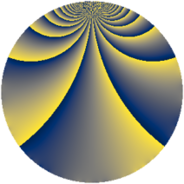# Properties

 Label 546.2.bgLevel $546$ Weight $2$ Character orbit 546.bg Rep. character $\chi_{546}(311,\cdot)$ Character field $\Q(\zeta_{6})$ Dimension $72$ Newform subspaces $2$ Sturm bound $224$ Trace bound $2$

# Related objects

## Defining parameters

 Level: $$N$$ $$=$$ $$546 = 2 \cdot 3 \cdot 7 \cdot 13$$ Weight: $$k$$ $$=$$ $$2$$ Character orbit: $$[\chi]$$ $$=$$ 546.bg (of order $$6$$ and degree $$2$$) Character conductor: $$\operatorname{cond}(\chi)$$ $$=$$ $$273$$ Character field: $$\Q(\zeta_{6})$$ Newform subspaces: $$2$$ Sturm bound: $$224$$ Trace bound: $$2$$ Distinguishing $$T_p$$: $$5$$

## Dimensions

The following table gives the dimensions of various subspaces of $$M_{2}(546, [\chi])$$.

Total New Old
Modular forms 240 72 168
Cusp forms 208 72 136
Eisenstein series 32 0 32

## Trace form

 $$72 q - 36 q^{4} + 8 q^{9} + O(q^{10})$$ $$72 q - 36 q^{4} + 8 q^{9} - 36 q^{16} + 28 q^{25} + 14 q^{30} - 16 q^{36} - 32 q^{42} - 32 q^{43} + 24 q^{49} - 6 q^{51} + 12 q^{52} - 72 q^{61} + 72 q^{64} - 48 q^{66} + 108 q^{75} + 40 q^{78} + 40 q^{79} - 40 q^{81} - 48 q^{82} - 48 q^{87} - 4 q^{91} - 144 q^{94} + O(q^{100})$$

## Decomposition of $$S_{2}^{\mathrm{new}}(546, [\chi])$$ into newform subspaces

Label Dim $A$ Field CM Traces $q$-expansion
$a_{2}$ $a_{3}$ $a_{5}$ $a_{7}$
546.2.bg.a $36$ $4.360$ None $$-18$$ $$0$$ $$0$$ $$0$$
546.2.bg.b $36$ $4.360$ None $$18$$ $$0$$ $$0$$ $$0$$

## Decomposition of $$S_{2}^{\mathrm{old}}(546, [\chi])$$ into lower level spaces

$$S_{2}^{\mathrm{old}}(546, [\chi]) \cong$$ $$S_{2}^{\mathrm{new}}(273, [\chi])$$$$^{\oplus 2}$$